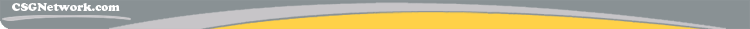# Test Grade Percentage Calculator

This calculator requires the use of Javascript enabled and capable browsers. This script simply tells you the percentage and test grade earned on a test. Input the points possible and the points given. The grade percentage is calculated by dividing the earned points by the total points possible. The formula is: Points earned / Points Possible, then that percentage is compared to the given scale. I added a custom scale option, and an option for plus and minus grades. Enjoy!

The grading scale used for letter grades is as follows:

 A+    100 B+    87-89 C+   77-79 D+   67-69 A     93-96 B     83-86 C    73-76 D     63-66 A-   90-92 B-   80-82 C-   70-72 D-   60-62 F    below  60

You may also want to try:
Grade Point Average (GPA) Calculator

 Test Grade Required Data Entry Use +/- grades Points Given Total Points Possible Calculated Results Grade Percentage: Letter Grade:
 Custom Scale Section Use custom scale Grade Starts At A+ A A- B+ B B- C+ C C- D+ D D-
Updated: 7/31/15

 Leave us a question or comment on FacebookSearch or Browse Our Site